When working with financial data, you will notice that currency values are automatically formatted by Excel to include a dollar sign.

This might not be the currency symbol you want to include. In some cases, you might not want the preceding currency symbol at all.

Fortunately, it’s possible to control your currency formatting on Excel quite easily, and there are multiple ways to do it.

In this tutorial, we will show you how to remove the dollar sign from your currency-formatted cells.

## Removing Dollar Sign by Using the Format Cells Dialog Box

If the dollar sign was added due to a currency format setting, then you can use this method to remove it.

Suppose you have a dataset as the one given below, and you want to remove all dollar signs from column B.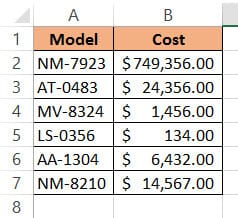Here are the steps you need to follow:

1. Select the cells containing the dollar signs that you want to remove
2. Right-click on your selection and click “Format Cells” from the popup menu.
3. This will open the Format Cells dialog box. Select the “Number” tab.
4. Under the Category list, select the ‘Currency’ option.
5. Click on the dropdown list next to “Symbol” and select the “None” option.
6. Click OK.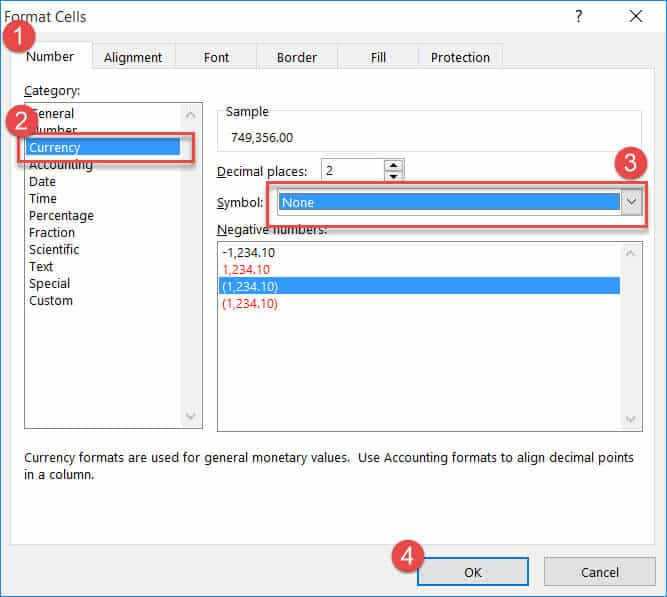This should remove the dollar symbol from all the selected cells.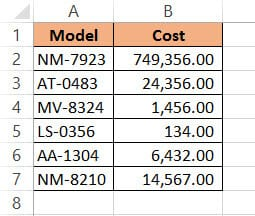## Removing Dollar Sign by Using a Formula

If the above method did not work, it might be because the dollar symbol was not added by formatting. Some people like to add the dollar symbol by converting the cell to text. You can easily tell if this was the case by checking for tell-tale signs:

• The cell would be aligned to the left, instead of right. Excel always aligns text to the left and numbers to the right within cells
• There would be a green triangle on the top left corner of the cell.
• If you click on the cell you can see a small leading apostrophe sign.
• You can also double check by typing the formula =istext in an empty cell and passing the reference to your cell. Then you press the return key. If you get the result as TRUE, it means your cell contains the text. If not, it means your cell does not contain text.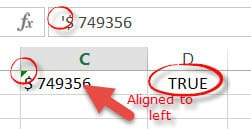If any of the above signs suggest that the cell is actually text, here’s how you can remove the dollar sign:

1. In a new column, type = RIGHT(B2,LEN(B2)-1). You can replace B2 with the reference to the first cell from which you want the dollar sign removed.
2. Press the Return key.
3. You will get the contents of the cell in question, with the dollar sign removed. This is assuming that the dollar sign was the first character in the text. You can copy this formula to the rest of the cells in the column by double-clicking the fill handle (at the bottom-right corner of the cell).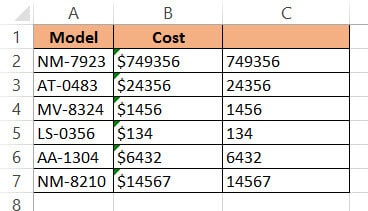### Explanation of the Formula

The formula that we used in the above method: = RIGHT(B2,LEN(B2)-1) extracts all the characters from cell B2, except for the first character.

The LEN() function is used to extract the total number of characters in B2. The formula subtracts 1 from this. So the final result gives all the characters starting from the right of the text, minus 1.

If the original cell (B2) has 6 characters, then LEN(B2)-1 gives 6 -1 =5.

The formula then returns RIGHT(B2, 5). This means “get the last 5 characters from cell B2”.

The first character of B2 was the dollar sign, so this gets removed.

Note: If your original cell had a different format, for example, if it had the dollar sign followed by a space followed by the actual number, then you would need to remove the first 2 characters. In such cases, replace LEN(B2)-1 to LEN(B2)-2.

## Removing Dollar Sign by using Find and Replace

If you find the cells having a consistent pattern, for example, if there’s a dollar sign followed by space (or some other character) followed by the actual number, you can use the Find and Replace feature.

Here’s how this method works:

1. Select the cells containing the dollar signs that you want to remove.
2. From the ‘Editing’ group, under the Home Tab, click on ‘Find and Select’ and click ‘Replace’ from the drop-down menu. Alternatively, you can just press CTRL+H from your keyboard.
3. This will open the ‘Find and Replace’ dialog box. In the ‘Find’ field, enter \$. (If there’s a space following the dollar sign, then enter \$ followed by a space character).
4. Leave the ‘Replace with’ field empty. This will make sure that all the dollar signs get replaced with a blank.
5. Click on the ‘Replace All’ button.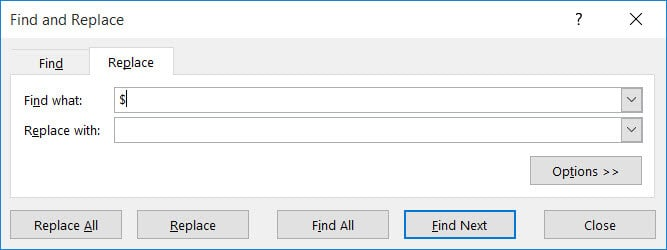All your dollar signs should get replaced in one go.

This method is great for cases in which you want to remove dollar signs from the entire sheet. In such cases, you don’t need to select any cells and just follow the steps from step 2 onwards.

You can also use this method to replace the dollar symbol with any other currency symbol.

## Removing Dollar Sign by Using the VALUE Function

If you are not sure about how the dollar sign was added, there’s a fool-proof way to extract just the value of the cells. This is by using the VALUE() function.

1. In a new column, type =VALUE() and within the brackets, put a reference to the first cell from which you want the dollar sign removed
2. Press the Return key.
3. You will find only the value of the cell in question, sans any formatting that had been applied. You can copy this formula to the rest of the cells in the column by double-clicking the fill handle.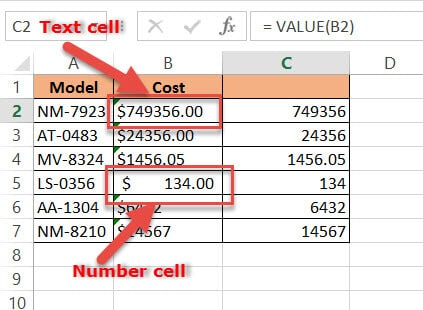By using the above method, you can retain both the original cells (containing the dollar sign) as well as the new cells (without the dollar signs).

The new cells will get updated every time the original cells are edited, and you can use this function’s result for further calculations.

## Conclusion

In this tutorial, we discussed some ways in which you can remove the dollar sign (\$) from cells in Excel. If the sheet was prepared by someone else, then there may have been multiple ways in which they inserted the \$ sign.

Some like to add it with formatting options, while others like to convert the cell to text.

We tried to show you different scenarios, and how you can remove the dollar sign in each scenario. We hope you found this tutorial useful.

Other Excel tutorials you may like: